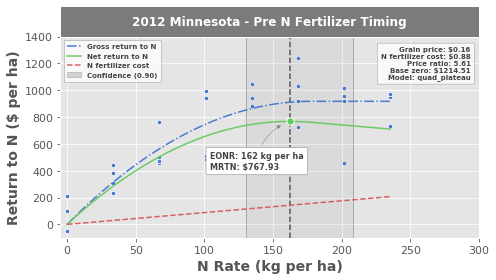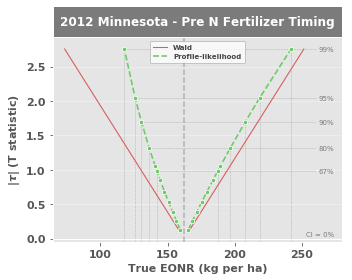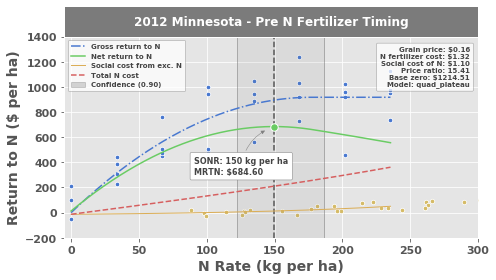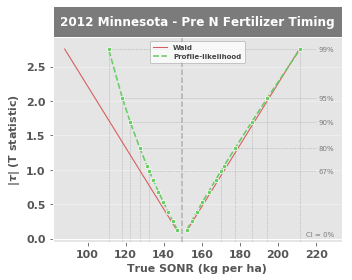# 2. Tutorial¶

Code for all the examples is located in your PYTHONPATH/Lib/site-packages/eonr/examples folder. With that said, you should be able to make use of EONR by following and executing the commands in this tutorial using either the sample data provided or substituting in your own data.

You will find the following code included in the tutorial.py or tutorial.ipynb (for Jupyter notebooks) files in your PYTHONPATH/Lib/site-packages/eonr/examples folder - feel free to load that into your Python IDE to follow along.

After installation, load Pandas and the EONR module in a Python interpreter:

:

import os
import pandas as pd
import eonr
print('EONR version: {0}'.format(eonr.__version__))

EONR version: 0.2.0


EONR uses Pandas dataframes to access and manipulate the experimental data.

:

df_data = pd.read_csv(os.path.join('data', 'minnesota_2012.csv'))
df_data

:

year location plot trt rep time_n rate_n_applied_kgha yld_grain_dry_kgha nup_total_kgha soil_plus_fert_n_kgha
0 2012 Minnesota 101 8 1 Pre 235.3785 12410.916200 198.759898 284.69590
1 2012 Minnesota 102 3 1 Pre 67.2510 10627.946000 147.971755 116.56840
2 2012 Minnesota 103 1 1 Pre 0.0000 7428.081218 98.769392 38.10890
3 2012 Minnesota 104 2 1 Pre 33.6255 9202.953180 111.440210 71.73440
4 2012 Minnesota 105 4 2 Pre 100.8765 10841.127180 142.663887 154.67730
5 2012 Minnesota 106 7 2 Pre 201.7530 10646.649330 178.802092 255.55380
6 2012 Minnesota 107 6 2 Pre 168.1275 12367.436000 186.053531 201.75300
7 2012 Minnesota 108 5 2 Pre 134.5020 13366.361700 196.737290 168.12750
8 2012 Minnesota 201 7 1 Pre 201.7530 14232.053480 228.775204 251.07040
9 2012 Minnesota 202 5 1 Pre 134.5020 14384.824980 226.006218 183.81940
10 2012 Minnesota 203 6 1 Pre 168.1275 13592.219290 182.423028 206.23640
11 2012 Minnesota 204 4 1 Pre 100.8765 14091.078390 187.745096 138.98540
12 2012 Minnesota 205 3 2 Pre 67.2510 10739.981390 133.470950 121.05180
13 2012 Minnesota 206 1 2 Pre 0.0000 8375.090921 109.460245 53.80080
14 2012 Minnesota 207 8 2 Pre 235.3785 13797.485850 195.932161 269.00400
15 2012 Minnesota 208 2 2 Pre 33.6255 9713.487469 113.903035 67.25100
16 2012 Minnesota 301 3 3 Pre 67.2510 12579.012170 180.812783 106.48075
17 2012 Minnesota 302 7 3 Pre 201.7530 13604.571780 208.724988 240.98275
18 2012 Minnesota 303 2 3 Pre 33.6255 10185.959390 121.505528 68.37185
19 2012 Minnesota 304 6 3 Pre 168.1275 14305.321460 204.391319 202.87385
20 2012 Minnesota 305 8 4 Pre 235.3785 13929.592020 186.775288 267.88315
21 2012 Minnesota 306 4 4 Pre 100.8765 10975.799250 147.057081 133.38115
22 2012 Minnesota 307 5 4 Pre 134.5020 11338.070290 148.348790 162.52325
23 2012 Minnesota 308 1 4 Pre 0.0000 5821.373521 68.791363 28.02125
24 2012 Minnesota 401 5 3 Pre 134.5020 13755.002370 198.844611 173.73175
25 2012 Minnesota 402 1 3 Pre 0.0000 9077.628329 122.207140 39.22975
26 2012 Minnesota 403 4 3 Pre 100.8765 13760.323240 181.734857 135.62285
27 2012 Minnesota 404 8 3 Pre 235.3785 14896.886860 227.454749 270.12485
28 2012 Minnesota 405 2 4 Pre 33.6255 10551.466010 144.282839 66.13015
29 2012 Minnesota 406 6 4 Pre 168.1275 15621.406530 227.056199 200.63215
30 2012 Minnesota 407 3 4 Pre 67.2510 10950.450720 146.628851 95.27225
31 2012 Minnesota 408 7 4 Pre 201.7530 13838.222530 210.340625 229.77425

## 2.4. Set column names (pre-init)¶

The table containing the experimental data must have a minimum of two columns:

• Nitrogen fertilizer rate

• Grain yield

EONR accepts custom column names. Just be sure to set them by either using EONR.set_column_names() or by passing them to EONR.calculate_eonr(). We will declare the names of the these two columns as they exist in the Pandas dataframe so they can be passed to EONR later:

:

col_n_app = 'rate_n_applied_kgha'
col_yld = 'yld_grain_dry_kgha'


Each row of data in our dataframe should correspond to a nitrogen rate treatment plot. It is common to have several other columns describing each treatment plot (e.g., year, location, replication, nitrogen timing, etc.). These aren’t necessary, but EONR will try pull information from “year”, “location”, and “nitrogen timing” for labeling the plots that are generated (as you’ll see towards the end of this tutorial).

## 2.5. Set units¶

Although optional, it is good practice to declare units so we don’t get confused:

:

unit_currency = '$' unit_fert = 'kg' unit_grain = 'kg' unit_area = 'ha'  These unit variables are only used for plotting (titles and axes labels), and they are not actually used for any computations. Because the initial parameter guess is critically important for fitting the model(s), there is logic built in that adjusts this intitial guess based on the units provided here. ## 2.6. Set economic conditions¶ EONR computes the Economic Optimum Nitrogen Rate for any economic scenario that we define. All that is required is to declare the cost of the nitrogen fertilizer (per unit, as defined above) and the price of grain (also per unit). Note that the cost of nitrogen fertilizer can be set to zero, and the Agronomic Optimum Nitrogen Rate will be computed. :  cost_n_fert = 0.88 # in USD per kg nitrogen price_grain = 0.157 # in USD per kg grain  ## 2.7. Initialize EONR¶ At this point, we can initialize an instance of EONR. Before doing so, we may want to set the base directory. EONR.base_dir is the default location for saving plots and data processed by EONR. If EONR.base_dir is not set, it will be set to be a folder named “eonr_tutorial” in the current working directory during the intitialization (to see your current working directory, type os.getcwd()). If you do not wish to use this as your current working directory, it can be passed to the EONR instance using the base_dir keyword. For demonstration purposes, we will set EONR.base_dir to what would be the default folder if nothing were passed to the base_dir keyword –> that is, we will choose a folder named “eonr_advanced_tutorial” in the current working directory (EONR will create the directory if it does not exist). And finally, to create an instance of EONR, pass the appropriate variables to EONR(): :  import os base_dir = os.path.join(os.getcwd(), 'eonr_tutorial') my_eonr = eonr.EONR(cost_n_fert=cost_n_fert, price_grain=price_grain, col_n_app=col_n_app, col_yld=col_yld, unit_currency=unit_currency, unit_grain=unit_grain, unit_fert=unit_fert, unit_area=unit_area, model=None, base_dir=base_dir)  Notice the model keywork argument was set to None (default=’quad_plateau’). This keyword is used to define which model to use to fit the experimental data. If None, EONR will try all supported models (i.e., as of v. 0.2.0, both ‘quad_plateau’ and ‘quadratic’ models are supported), and use the model that fits the data best as determined by the highest $$\text{r}^2$$. ## 2.8. Calculate the EONR¶ With my_eonr initialized as an instance of EONR, we can now calculate the economic optimum nitrogen rate by calling the calculate_eonr() method and passing the dataframe with the loaded data: :  my_eonr.calculate_eonr(df_data)   Computing EONR for Minnesota 2012 Pre Cost of N fertilizer:$0.88 per kg
Price grain: $0.16 per kg Fixed costs:$0.00 per ha
Economic optimum N rate (EONR): 162.3 kg per ha [130.5, 207.8] (90.0% confidence)
Maximum return to N (MRTN): $767.93 per ha  It may take a few seconds to run, but it will take much longer if you choose to compute the bootstrap confidence intervals in addition to the profile-likelihood confidence intervals. Please see the Advanced tutorial describing how to compute the bootstrap CIs (EONR does not run the bootstrap CIs by default). And that’s it! The economic optimum for this dataset and economic scenario was 162 kg nitrogen per ha (with 90% confidence bounds at 131 and 208 kg per ha) and resulted in a maximum net return of nearly$770 per ha.

Notice the $$\text{r}^2$$ for the quadratic-plateau model was greater than that of the quadratic model, so the quadratic-plateau model was used (the model used is recorded in EONR.df_results and will be displayed in the plot legend - see below).

## 2.9. Plotting the EONR¶

This is great, but of course it’d be useful to see our data and results plotted. Do this by calling the plot_eonr() module and (optionally) passing the minimum/maximum values for each axis:

:

my_eonr.plot_eonr(x_min=-5, x_max=300, y_min=-100, y_max=1400)• The blue points are experimental data (yield value in \$per ha as a function of nitrogen rate) • The blue line is the best-fit quadratic-plateau model representing gross return to nitrogen • The red line is the cost of nitrogen fertilizer • The green line is the difference between the two and represents the net return to nitrogen • The green point is the Economic Optimum Nitrogen Rate (EONR) • The transparent grey box surrounding the EONR/MRTN (green point) illustrates the 90% confidence intervals The EONR is the point on the x-axis where the net return curve (green) reaches the maximum return. The return to nitrogen at the EONR is the Maximum Return to Nitrogen (MRTN), indicating the profit that is earned at the economic optimum nitrogen rate. Notice the economic scenario (i.e., grain price, nitrogen fertilizer cost, etc.) and the “Base zero” values in the upper right corner describing the assumptions of EONR calculatioon. Base zero refers to the initial y-intercept of the gross return model (this setting can be turned on/off by setting EONR.base_zero to True or False. See the Advanced tutorial and the API for more information. ## 2.10. Accesing complete results¶ All results (e.g., EONR, MRTN, $$\text{r}^2$$ and root mean squared errors from best-fit models, confidence intervals, etc.) are stored in the EONR.df_results dataframe: :  my_eonr.df_results  :  price_grain cost_n_fert cost_n_social costs_fixed price_ratio unit_price_grain unit_cost_n location year time_n ... mrtn grtn_r2_adj grtn_rmse grtn_max_y grtn_crit_x grtn_y_int scn_lin_r2 scn_lin_rmse scn_exp_r2 scn_exp_rmse 0 0.157 0.88 0.0 0.0 5.605096$ per ha $per kg Minnesota 2012 Pre ... 767.930477 0.728926 181.225993 917.43358 177.439985 0.002964 None None None None 1 rows × 33 columns See the Advanced tutorial for a description of every column. ## 2.11. Visualizing all confidence intervals¶ By default, the confidence intervals (CIs) are calculated at many alpha levels. Noting that $$\text{CI} = 1-\alpha$$, let’s plot the Wald CIs and profile-likelihood CIs for a range of $$\alpha$$ values. EONR.plot_modify_size() can be called to make basic adjustments to the figure size. Because EONR.fig_tau.fig is a Matplotlib figure object, you’ll be able to make any customizations within the Matplotlib API as well. :  my_eonr.plot_tau() my_eonr.fig_tau = my_eonr.plot_modify_size(fig=my_eonr.fig_tau.fig, plotsize_x=5, plotsize_y=4.0)This plot shows the lower and upper confidence intervals of the True EONR (True EONR refers to the actual EONR value, which is not actually known due to uncertainty in the dataset). At 0% confidence, the True EONR is the maximum likelihood value, but as we increase the confidence level from 67%, 80%, 90%, 95%, and 99%, the statistical range of the True EONR widens. In general, the profile-likelihood CIs are considered the most accurate of the three methods because they reflect the actual, often asymmetric, uncertainty in a parameter estimate Cook & Weisberg, 1990. ## 2.12. Accessing complete CI results¶ All data relating to the calculation of the CIs are saved in the EONR.df_ci dataframe: :  my_eonr.df_ci  :  run_n location year time_n price_grain cost_n_fert cost_n_social price_ratio f_stat t_stat level wald_l wald_u pl_l pl_u boot_l boot_u opt_method_l opt_method_u 0 1 Minnesota 2012 Pre 0.157 0.88 0.0 5.605096 0.000000 0.000000 0.000 NaN NaN NaN NaN NaN NaN N/A N/A 1 1 Minnesota 2012 Pre 0.157 0.88 0.0 5.605096 0.016070 0.126767 0.100 158.247550 166.432039 159.432270 165.322890 NaN NaN Nelder-Mead Nelder-Mead 2 1 Minnesota 2012 Pre 0.157 0.88 0.0 5.605096 0.065374 0.255684 0.200 154.085886 170.593703 156.557488 168.432973 NaN NaN Nelder-Mead Nelder-Mead 3 1 Minnesota 2012 Pre 0.157 0.88 0.0 5.605096 0.151446 0.389161 0.300 149.777004 174.902584 153.670496 171.730580 NaN NaN Nelder-Mead Nelder-Mead 4 1 Minnesota 2012 Pre 0.157 0.88 0.0 5.605096 0.281127 0.530214 0.400 145.223559 179.456029 150.721517 175.297880 NaN NaN Nelder-Mead Nelder-Mead 5 1 Minnesota 2012 Pre 0.157 0.88 0.0 5.605096 0.466549 0.683044 0.500 140.289960 184.389628 147.647498 179.255088 NaN NaN Nelder-Mead Nelder-Mead 6 1 Minnesota 2012 Pre 0.157 0.88 0.0 5.605096 0.729644 0.854192 0.600 134.765004 189.914585 144.357366 183.796319 NaN NaN Nelder-Mead Nelder-Mead 7 1 Minnesota 2012 Pre 0.157 0.88 0.0 5.605096 0.969292 0.984526 0.667 130.557584 194.122004 141.960754 187.330059 NaN NaN Nelder-Mead Nelder-Mead 8 1 Minnesota 2012 Pre 0.157 0.88 0.0 5.605096 1.113663 1.055302 0.700 128.272818 196.406771 140.698855 189.275802 NaN NaN Nelder-Mead Nelder-Mead 9 1 Minnesota 2012 Pre 0.157 0.88 0.0 5.605096 1.719858 1.311434 0.800 120.004454 204.675134 136.361782 196.472716 NaN NaN Nelder-Mead Nelder-Mead 10 1 Minnesota 2012 Pre 0.157 0.88 0.0 5.605096 2.887033 1.699127 0.900 107.489043 217.190545 130.457577 207.827070 NaN NaN Nelder-Mead Nelder-Mead 11 1 Minnesota 2012 Pre 0.157 0.88 0.0 5.605096 4.182964 2.045230 0.950 96.316254 228.363335 125.804436 218.441868 NaN NaN Nelder-Mead Nelder-Mead 12 1 Minnesota 2012 Pre 0.157 0.88 0.0 5.605096 7.597663 2.756386 0.990 73.358903 251.320685 117.769597 241.793290 NaN NaN Nelder-Mead Nelder-Mead ## 2.13. Adjusting the economic scenario¶ These results were calculated for a specific economic scenario, but the cost of fertilizer and price of grain can be adjusted to run EONR for another economic scenario. Just adjust the economic scenario by passing any of: • cost_n_fert • price_grain • cost_n_social to EONR.update_econ(): :  cost_n_fert = 1.32 # adjusted from$0.88 per kg nitrogen
my_eonr.update_econ(cost_n_fert=cost_n_fert)


## 2.14. Environmental observations¶

You’ll notice above that we can pass the cost_n_social variable to EONR.update_econ(). This is becuase EONR will calculate the Socially Optimum Nitrogen Rate (SONR) if certain environmental data are available. For more information about the SONR, refer to the Background chapter.

In the same way that cost_n_fert was adjusted in the previous code, cost_n_social will be set (for the first time):

:

cost_n_social = 1.10 # in USD per kg nitrogen
my_eonr.update_econ(cost_n_social=cost_n_social)


## 2.15. Calculate mineralization¶

You may have noticed that the loaded data for this tutorial contains columns for nitrogen uptake (“nup_total_kgha”) and available nitrogen (“soil_plus_fert_n_kgha”). This data can be used to calculate the approximate net mineralization for the zero rate, which can be assumed to consistent across all rates. Total crop available nitrogen can then be assumed to be the sum of fertilizer, soil, and net mineralized nitrogen. We can then calculate the SONR as long as the column names are correctly set.

The column names were set for nitrogen fertilizer rate (col_n_app) and grain yield (col_yld) during the initialization of EONR, but they haven’t been set for the nitrogen uptake or available nitrogen columns. This can be done (even after initilization of EONR) using EONR.set_column_names():

:

def calc_mineralization(df_data, units_fert='kgha'):
'''
Calculates mineralization and adds "crop_available_n" to df
'''
df_trt0 = df_data[df_data['rate_n_applied_kgha']==0].copy()
df_trt0['mineralize_n'] = (df_trt0['nup_total_kgha'] -
df_trt0['soil_plus_fert_n_kgha'])
trt0_mineralize = df_trt0['mineralize_n'].mean()
crop_n_label = 'crop_n_available_' + units_fert
df_data[crop_n_label] = df_data['soil_plus_fert_n_kgha'] + trt0_mineralize
return df_data

df_data = calc_mineralization(df_data)

col_crop_nup = 'nup_total_kgha'
col_n_avail = 'crop_n_available_kgha'

my_eonr.set_column_names(col_crop_nup=col_crop_nup,
col_n_avail=col_n_avail)


EONR simply subtracts end of season total nitrogen uptake from available nitrogen to get net crop nitrogen use, which is subsequently used to calculate the SONR.

## 2.16. Run EONR for the socially optimum rate¶

Then simply run EONR.calculate_eonr() again to calculate the SONR for the updated economic scenario:

:

my_eonr.calculate_eonr(df_data)


Computing SONR for Minnesota 2012 Pre
Cost of N fertilizer: $1.32 per kg Price grain:$0.16 per kg
Fixed costs: $0.00 per ha Social cost of N:$1.10 per kg
Socially optimum N rate (SONR): 149.6 kg per ha [122.4, 186.3] (90.0% confidence)
Maximum return to N (MRTN): $684.60 per ha  The new results are appended to the EONR.df_results dataframe: :  my_eonr.df_results  :  price_grain cost_n_fert cost_n_social costs_fixed price_ratio unit_price_grain unit_cost_n location year time_n ... mrtn grtn_r2_adj grtn_rmse grtn_max_y grtn_crit_x grtn_y_int scn_lin_r2 scn_lin_rmse scn_exp_r2 scn_exp_rmse 0 0.157 0.88 0.0 0.0 5.605096$ per ha $per kg Minnesota 2012 Pre ... 767.930477 0.728926 181.225993 917.43358 177.439985 0.002964 None None None None 1 0.157 1.32 1.1 0.0 15.414013$ per ha $per kg Minnesota 2012 Pre ... 684.596933 0.728926 181.225993 917.43358 177.439985 0.002964 0.777106 139.314 0.836099 21.1184 2 rows × 33 columns EONR.plot_eonr() and EONR.plot_tau() can be called again to plot the new results: :  my_eonr.plot_eonr(x_min=-5, x_max=300, y_min=-200, y_max=1400) my_eonr.plot_tau() fig_tau = my_eonr.plot_modify_size(fig=my_eonr.fig_tau.fig, plotsize_x=5, plotsize_y=4.0)Notice the added data in the nitrogen response plot: • The gold points represent net crop nitrogen use (expressed as a \$ amount based on the value set for cost_n_social)

• The gold line is the best-fit exponential model representing net crop nitrogen use (EONR fits both a linear and exponential model for this, then uses whichever has a higher $$\text{r}^2$$)

## 2.17. Saving the data¶

The results generated by EONR can be saved to the EONR.base_dir using the Pandas df.to_csv() function. A folder will be created in the base_dir whose name is determined by the current economic scenario of my_eonr (in this case “social_154_1100”, corresponding to cost_n_social > 0, price_ratio = 15.4, and cost_n_social = 1.10 for “social”, “154”, and “1100” in the folder name, respectively):

:

print(my_eonr.base_dir)

my_eonr.df_results.to_csv(os.path.join(os.path.split(my_eonr.base_dir), 'tutorial_results.csv'), index=False)
my_eonr.df_ci.to_csv(os.path.join(os.path.split(my_eonr.base_dir), 'tutorial_ci.csv'), index=False)

F:\nigo0024\Documents\GitHub\eonr\docs\source\eonr_tutorial\social_154_1100


Upon generating figures using EONR.plot_eonr() or EONR.plot_tau(), the matplotlib figures are stored to the EONR class. They can be saved to file by using EONR.plot_save():

:

fname_eonr_plot = 'eonr_mn2012_pre.png'
fname_tau_plot = 'tau_mn2012_pre.png'

my_eonr.plot_save(fname=fname_eonr_plot, fig=my_eonr.fig_eonr)
my_eonr.plot_save(fname=fname_tau_plot, fig=my_eonr.fig_tau)